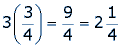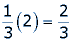Math Central - mathcentral.uregina.caMath Beyond Schoolreturn to topMaking Enough: Working with Recipes Most recipes give guidelines as to how much a single batch will produce.  But what if you want more?  It seems too time consuming to mix up another batch.  What if the recipe makes only one dozen cupcakes and you need three dozen?  Clearly, three dozen is three times more than 1 dozen, so we can multiply all the ingredients by three to make a larger batch.  It is also important to understand how to multiply fractions.  If the cupcake recipe calls for ¾ cup of milk and we want to triple it, we need to know that:So, we will need 2 and 1/4 cups of milk to make three dozen cupcakes. This knowledge of fractions is also helpful when we need to make our batch smaller.  For example, recipe guidelines approximate that each batch will yield 6 dozen cookies.  But, my family is small and I only want 2 dozen cookies.  First, we need to see the relationship between 2 and 6.  We can see that 2 dozen is one third (1/3) of 6 dozen because 2 x 3 = 6.  That means that in order to make only 2 dozen cookies, we will need to use one third of each ingredient.  So, if the recipe asks for 2 teaspoons of baking powder, we will only need 2/3 of a teaspoon, sinceIf we do not have a measuring spoon that is equal to 2/3 teaspoon, we may need to use 1/3 twice, or estimate using ¼.  When recipes indicate how much a particular batch will make, they give a general amount of food.  If we are cooking for a group, we need to estimate how much each person will eat and make appropriate amounts of the particular item.  For example, if a package of spaghetti makes 1L of cooked spaghetti, will we have enough to feed six people with one package?  If not, how much of a second package will we have to use?  First, we need to estimate how much each person will eat.  We can guess that each person will eat 1 cup of spaghetti, which is 237mL.  For convenience sake, we can round this to 250mL.  That means that six people will eat 1500mL of spaghetti. If 1L=1000mL, we know that we will need to make one whole package, plus half of the second package to ensure that everyone has enough to eat.
 Math Central is supported by the University of Regina and The Pacific Institute for the Mathematical Sciences.about math central :: site map :: links :: notre site français